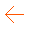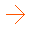Digital potentiometer: AD5171 Master reader Master writer Slave receiver Slave sender Ultrasonic ranger: SFR08/SFR10 Compass heading: HMC6352 sparkfun Compass heading with tilt compensation: HMC6352 sparkfun Real time clock: DS1307 sparkfun Temperature and barometric pressure: Sparkfun BOSCH BMP085 Temperature: Sparkfun tmp102 Barometric pressure: SCP1000 MLX90609 Gyroscope: Sparkfun Servo moving Multiple servos Servo & potentiometer Hello Matrix Sprite animation Printing data to parallel LCD display Encoder read Storing data EEPROM data variables All inputs Analog inputs Strings accept and echoing Wire & Firmata Old Standard protocol Servo & Firmata Analog input/output pins Digital input/output pins Standard protocol Software serial port test Two ports Stepper move Basic positioning Course to destination Determining speed Distance to destination NMEA Sentence Button object Event API for buttons Button polling technique Constrained variables Hello fluent print LED state machine Hello Hashmap Custom Keypad Dynamic Keypad Event Keypad Hello Keypad Blink Loop Hello Menu Hello OSC (Open Sound Control) Basic communication Check string Copy string Hello Password Password Keypad Serial Monitor PotControl LED Pot Demo Delayed Signal Hello Supervisor Hello Timed Action Three Examples At OnceThis example is for Wiring version 1.0 build 0100+. If you have a previous version, use the examples included with your software. If you see any errors or have comments, please let us know.

RTC Control v.01 by John Vaughters Credit to: Maurice Ribble - http://www.glacialwanderer.com/hobbyrobotics for RTC DS1307 code

With this code you can set the date/time, retreive the date/time and use the extra memory of an RTC DS1307 chip. The program also sets all the extra memory space to 0xff. Serial Communication method with the Arduino that utilizes a leading CHAR for each command described below. Commands: T(00-59)(00-59)(00-23)(1-7)(01-31)(01-12)(00-99) - T(sec)(min)(hour)(dayOfWeek)(dayOfMonth)(month)(year) - T Sets the date of the RTC DS1307 Chip. Example to set the time for 02-Feb-09 @ 19:57:11 for the 3 day of the week, use this command - T1157193020209 Q(1-2) - (Q1) Memory initialization (Q2) RTC - Memory Dump On Wiring v1 boards the SCL and SDA pins are: 0 and 1 On Wiring S board the SCL and SDA pins are: 8 and 9```#include <Wire.h>

int clockAddress = 0x68;  // This is the I2C address
int command = 0;  // This is the command char, in ascii form, sent from the serial port
long previousMillis = 0;  // will store last time Temp was updated
byte second, minute, hour, dayOfWeek, dayOfMonth, month, year;
byte test;

// Convert normal decimal numbers to binary coded decimal
byte decToBcd(byte val)
{
return ( (val/10*16) + (val%10) );
}

// Convert binary coded decimal to normal decimal numbers
byte bcdToDec(byte val)
{
return ( (val/16*10) + (val%16) );
}

// 1) Sets the date and time on the ds1307
// 2) Starts the clock
// 3) Sets hour mode to 24 hour clock
// Assumes you're passing in valid numbers,
// Probably need to put in checks for valid numbers.
void setDateDs1307()
{
// Use of (byte) type casting and ascii math to achieve result.
second = (byte) ((Serial.read() - 48) * 10 + (Serial.read() - 48));
minute = (byte) ((Serial.read() - 48) *10 +  (Serial.read() - 48));
hour  = (byte) ((Serial.read() - 48) *10 +  (Serial.read() - 48));
dayOfWeek = (byte) (Serial.read() - 48);
dayOfMonth = (byte) ((Serial.read() - 48) *10 +  (Serial.read() - 48));
month = (byte) ((Serial.read() - 48) *10 +  (Serial.read() - 48));
year= (byte) ((Serial.read() - 48) *10 +  (Serial.read() - 48));
Wire.write(byte(0x00));
Wire.write(decToBcd(second));  // 0 to bit 7 starts the clock
Wire.write(decToBcd(minute));
Wire.write(decToBcd(hour));    // If you want 12 hour am/pm you need to set
// bit 6 (also need to change readDateDs1307)
Wire.write(decToBcd(dayOfWeek));
Wire.write(decToBcd(dayOfMonth));
Wire.write(decToBcd(month));
Wire.write(decToBcd(year));
Wire.endTransmission();
}

// Gets the date and time from the ds1307 and prints result
void getDateDs1307() {
// Reset the register pointer
Wire.write(byte(0x00));
Wire.endTransmission();

// A few of these need masks because certain bits are control bits
second     = bcdToDec(Wire.read() & 0x7f);

// Need to change this if 12 hour am/pm
hour       = bcdToDec(Wire.read() & 0x3f);

Serial.print(hour, DEC);
Serial.print(":");
Serial.print(minute, DEC);
Serial.print(":");
Serial.print(second, DEC);
Serial.print("  ");
Serial.print(month, DEC);
Serial.print("/");
Serial.print(dayOfMonth, DEC);
Serial.print("/");
Serial.print(year, DEC);

}

void setup() {
Wire.begin();
Serial.begin(57600);
}

void loop() {
if (Serial.available()) {  // Look for char in serial que and process if found
if (command == 84) {      //If command = "T" Set Date
setDateDs1307();
getDateDs1307();
Serial.println(" ");
}
else if (command == 81) {  //If command = "Q" RTC1307 Memory Functions
delay(100);
if (Serial.available()) {

// If command = "1" RTC1307 Initialize Memory - All Data will be set to 255 (0xff).
// Therefore 255 or 0 will be an invalid value.
if (command == 49) {

// 255 will be the init value and 0 will be cosidered an error that
// occurs when the RTC is in Battery mode.

// Set the register pointer to be just past the date/time registers.
Wire.write(byte(0x08));
for (int i = 1; i <= 27; i++) {
Wire.write(byte(0xff));
delay(100);
}
Wire.endTransmission();
getDateDs1307();
Serial.println(": RTC1307 Initialized Memory");
}
else if (command == 50) {      //If command = "2" RTC1307 Memory Dump
getDateDs1307();
Serial.println(": RTC 1307 Dump Begin");
Wire.write(byte(0x00));
Wire.endTransmission();
for (int i = 1; i <= 64; i++) {
Serial.print(i);
Serial.print(":");
Serial.println(test, DEC);
}
Serial.println(" RTC1307 Dump end");
}
}
}
Serial.print("Command: ");
Serial.println(command);  // Echo command CHAR in ascii that was sent
}

command = 0;  // reset command
delay(100);
}
```